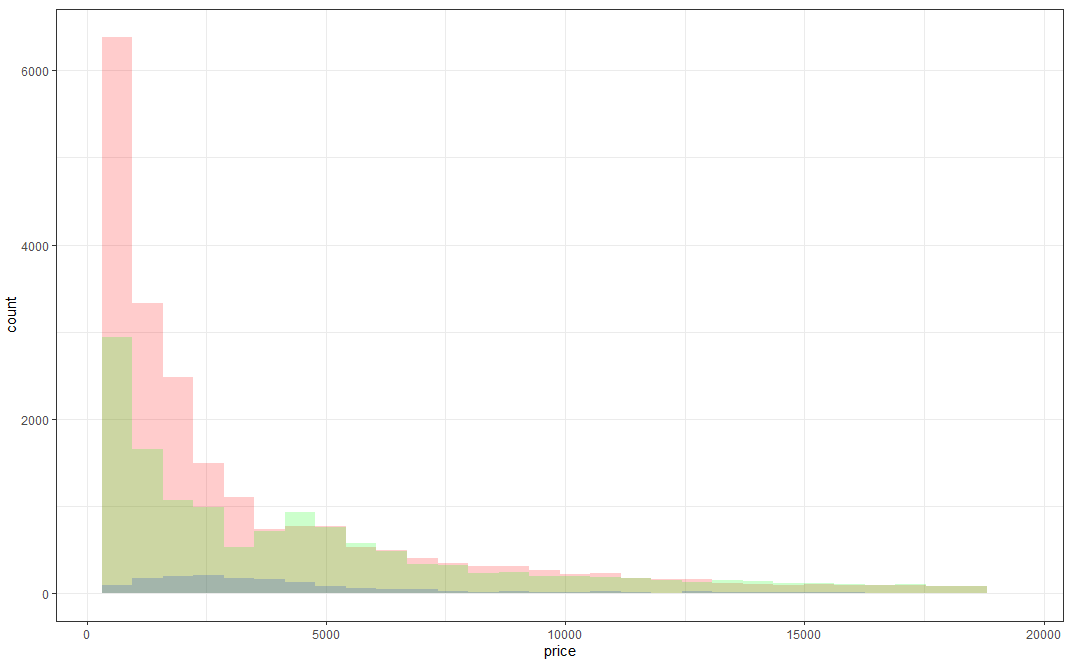2 views

I am new to R and am trying to plot 3 histograms onto the same graph. Everything worked fine, but my problem is that you don't see where 2 histograms overlap - they look rather cut off: Histogram

When I make density plots, it looks perfect: each curve is surrounded by a black frame line, and colors look different where curves overlap: Density Plot

Can someone tell me if something similar can be achieved with the histograms in the 1st picture? This is the code I'm using:

lowf0\$utt<-'low f0'

mediumf0\$utt<-'medium f0'

highf0\$utt<-'high f0'

histogram<-rbind(lowf0,mediumf0,highf0)

ggplot(histogram, aes(f0, fill = utt)) + geom_histogram(alpha = 0.2)

Thanks in advance for any useful tips!

by

To create three overlaying histograms, you can create three histograms separately with alpha blending, and then use separate calls to the geom_histogram function to plot them with their respective data and fill.

For example:

data(diamonds)

ggplot(diamonds,aes(x=price)) +

theme_bw()+

geom_histogram(data=subset(diamonds,cut == "Ideal"),fill = "red", alpha = 0.2) +

geom_histogram(data=subset(diamonds,cut == "Fair"),fill = "blue", alpha = 0.2) +

geom_histogram(data=subset(diamonds,cut == "Premium"),fill = "green", alpha = 0.2)

Output: